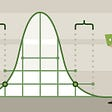# Welcome back!

This is a series of blogs meant to be an introduction into statistics for those who are interested in learning Data Science and Machine Learning, but may not have had any exposure to statistics.

In “Statistics… Lesson 1” I covered some of the basics of what statistics is and how its related to data science and machine learning — you can find it here:

Here in Lesson 2, we will continue the discussion where we left off.

# A Short Recap Before We Dive In

There are a few key points that I believe are important to keep top of mind while you learn statistics. I am going to summarize below.

There are two types of statistics — Descriptive and Inferential. Descriptive Statistics allows us to describe the data we are considering. Inferential Statistics allows us to propose conclusions based on the data.

A population is an entire set of data. A sample is a smaller, subset of the population. For the most part in statistics, we work with samples. A measure of a sample is called a statistic.

There are three types of data that can be considered:

(1) Interval Data

(2) Nominal Data

(3)Ordinal Data

Interval Data are numerical observations, such as your age or how many steps you took in a day.

Nominal Data are categorical observations, such as the color of your shirt or whether you walk, bike or take transit to work or school.

Ordinal Data is categorical data that can be placed into a particular order.

# Graphical Techniques

Graphs are a great tool to express and consider a set of data. Depending on the type of data you are working with, as discussed above, the graphical technique you choose to use will differ.

If you recall, Nominal Data, consists of categorical observations — in other words, data that can be placed into specific categories based on the observation. An example would be a type of car — Honda, Ford, Volkswagen or Hyundai. The observation of the type of car can be placed into one of those categories.

Nominal Data only has one calculation that can be performed, which is counting the frequency of an observation. Frequency is a way of saying how many times did we observe something. Continuing with the example above, you may have observed, out of 10 cars in total, 4 Hondas, 2 Fords and 3 Volkswagens and 1 Hyundai. The frequency of observing a Honda is 4 out of 10.

To represent this data visually we would create either a bar chart or a pie chart.

A bar chart allows us to display the frequencies that we observe. 4 out of 10 cars were Honda, while 2 out of 10 were Fords. An example is below.

A pie chart provides for a different consideration of the data.

Where a bar chart considers frequency, a pie chart considers the proportions of an individual observation within the entire set of observations.

Let’s unpack that statement for clarity.

A pie chart is a way to visualize percentages. 40% of the cars observed were Hondas. The proportions displayed in a pie chart are the percentages. It is the same data as a bar chart, but looked at in a different way. See the example pie chart below.

As you can see, each slice of the pie represents the size of each observation out of the whole. The majority of the observations of car models were Hondas, so the “Honda” slice is the largest.

The examples above presented Nominal Data visually. But how would you know when to use a bar chart versus a pie chart? Let’s discuss.

A bar chart is best for when you are considering nominal — or ordinal — data. If you recall, ordinal data is simply nominal data that can be put into a particular order. A bar chart is used when the data you have collected represents frequencies — or how many times you observed an event out of a total number of observations. Simply, frequencies are just plain numbers like 4 out of 10.

A pie chart is best for only nominal data (not ordinal). A pie chart would be used when proportions are being considered, perhaps when considering sets of samples. This would likely be a case where percentages are being considered. For instance, in a sample set, 40% of the observations were X, 30% were Y and 30% were Z. The pie chart will provide a visual representation of the distributions of the proportions as part of a whole.

If you recall from Lesson 1, interval data allows for the broadest set of calculations to be performed. On a set of interval data, one could calculate the mean, median, mode, standard deviation and variance.

The data is recorded in a set of intervals — called classes — that make up the set of observations. The classes (intervals) are basically data broken up into ranges.

Let’s consider an example.

A utility pulls data on the monthly billings to customers. The charges range from \$80 per month to \$500 per month depending on usage. This data would first be broken up into classes (intervals) to organize the data for further study.

In this case, perhaps the bills are broken up as follows:

Let’s pause here for a moment.

There is an important concept here that needs to be explored. The table pictured above is broken up in a particular manner. There is a simple equation that determines how to create what is known as the class width, or in other words the range between each set of recorded billings.

Class width is determined by the following:

Class Width = (Largest Observation — Smallest Observation)/ # of Classes

I decided to divide this data into 5 classes. To determine how the records are arranged within the 5 classes, I determined the class width (or, basically, the range each interval would represent) using the equation above.

The largest observation (the highest bill issued) was \$500. I subtracted from the smallest observation (the lowest bill issued) and divided by 5 (the number of classes I chose to represent the intervals). This gave me 84. I rounded down, and divided each interval into ranges of \$80.

To graph interval data, we use a special type of graph called a Histogram. A histogram looks similar to a bar chart, but they are not at all the same thing and you must be careful to differentiate the two.

Determining class width can be an art as much as a science. You may find there is some trial and error in determining the number of classes to best represent the data so that the histogram provides the greatest value. Why? Well, you would not want to use a width that is too small or too large.

The shapes of histograms is important.

A histogram can be symmetrical, like the below, meaning the data is not favored to frequencies observed in classes on the left or right side.

A histogram can be positively skewed, meaning the data shows the observed frequencies are higher in classes on the left hand side:

A histogram may be negatively skewed, meaning the data shows the observed frequencies are higher in classes on the right hand side:

We will end Lesson 2 with a short discussion of class relative frequency, and its relation to histograms.

When considering a data set, one may find it preferable to show the relative frequency (or the proportion) of observations falling into each class, rather than the frequencies individually.

Looking at our example of utility bills, it may be more useful to consider how many bills fall between \$160 and \$240 rather than how many are priced at \$160 exactly.

Relative frequencies should be used when looking at population relative frequencies, when comparing two or more histograms or when the number of observations included in the samples being studied are different.

To calculate the ‘Class Relative Frequency’ is as follows:

Class Relative Frequency = Class Frequency / Total number of observations

Lesson 3 will cover Scatter Plots and Measures of Central Tendency!

--

--

--

Avid learner pursuing data science and AI

Love podcasts or audiobooks? Learn on the go with our new app.

## The Role of Decision Tree in Data Mining## Oracle Analytics Cloud (OAC) & Remote Data Gateway (RDG)## Point of View — location technology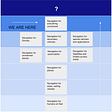## A/B testing with Random Forest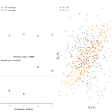## How Your Nonprofit Can Find the Time to Be More Data-Driven## Review: Trimps-Soushen — Winner in ILSVRC 2016 (Image Classification)## DATA LAKE: UNDERSTAND FROM A SENIOR MANAGER LENS## You are underutilizing shap values — feature groups and correlations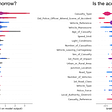## A. Jonathan DeCaria

Avid learner pursuing data science and AI

## Bias and Variance Trade Off…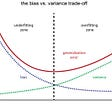## Ensemble techniques — Improvement over traditional Decision Trees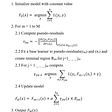## 7 Probability distributions you should know as a Data Scientist.